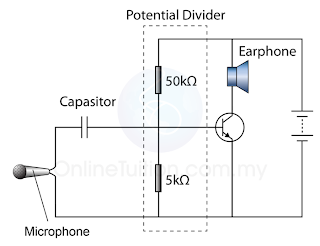# Transistor as a Current Amplifier

### Transistor as an Amplifier

1. The major application of a transistor is as a current amplifier.
2. A transistor can be used to amplify (‘magnify’) current changes because a small change in base current produces a large change in collector current.
3. A simple transistor amplifier circuit is shown in Figure 1 below.(Figure 1)
1. The graph in Figure 2 below shows the relationship between the base current and the collector current. From the graph, we can conclude that the collector current is directly proportional to the base current.(Figure 2: The collector current is directly proportional to the emitter current)
1. Since the small change in the base current IB results in a big change in the collector current, IC, the transistor, therefore, function as a current amplifier.
2. The ratio IC/IB is called the amplification factor.3. Figure 3 below shows another amplification circuit. In this case, however, the base current is varying because of the small alternating voltage produced by the microphone.(Figure 3)
1. The small changes in base current cause much larger changes in collector current.
2. The collector circuit includes an earphone through which you would hear an amplified version of the original sound.
3. The input capacitor passes on current changes from the microphone but blocks the steady current which might otherwise flow through the microphone from the potential divider. Such a current would upset the biasing effect of the potential divider.